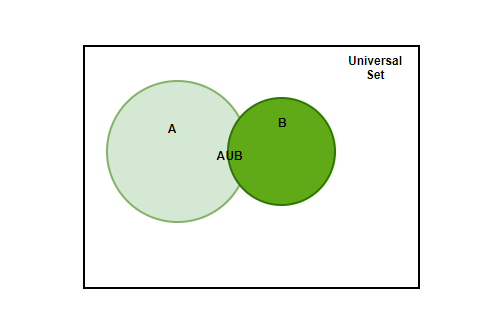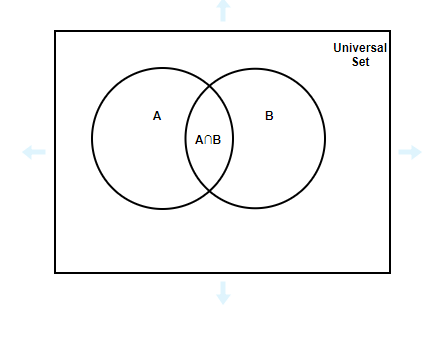Open in App
Not now

# Union and Intersection of Sets

• Last Updated : 20 Dec, 2022

Sets are the well-defined collection of data/objects. The data/objects belong to the same group but each data differs from one another. For instance, if the word “application” has to be put in a Set, it will look something like, Set A= {a, p, l, i, c, t, o, n}, the letters which are repeated in the word, like “p”, “a”, “i” are only written once since they are the same elements repeated.

### Types of Sets

There are different kinds of sets based on what type of element and how many elements are present in the set, there can be 5 elements, or 1 element, or finite but too many elements, or no element at all in the set. Let’s take a look at the types,

• Singular Set⇢ A set having only one element. For example, A= {1}, B= {e}, C= {a: a∈N, 5<a>7}.
• Null Set⇢ Null sets are those in which no element is present. Null sets are also known as Empty set or Void set. Null sets exist in order to explain certain parameters, for example, if a question asks to put the numbers in a set that are greater than 8 and less than 6, are there any numbers existing? No. Therefore, in such a case, Null set ⇢ {}/∅ is used. Null set is also important as it is a part of every set, the subset of each and every set.
• Finite Set ⇢ Finite sets have a finite number of elements, the amount of elements does not play an important role, as long as there are a finite number of elements present in the set, the set will be called
• a Finite set. For example, A= {a, e, i, o, u}, B= {1, 3, 5, 7, 9….95, 97, 99}.
• Infinite Set ⇢ Infinite sets are those in which an infinite number of elements are present, it is possible to have the infinite number of elements in the set. For instance, if someone asks about the natural numbers, and it is required to put them in a set, the natural numbers start from 1, but then they are endless, they go up to infinity, therefore, the elements in the set of all-natural numbers will be infinity. Infinite sets are denoted with dots at the end of the last element written to show that it goes up to infinity. For example, A= {2, 4, 6, 8, 10, 12….}

### Set Operations

In Set theory, it is very common to see two or more two sets showing some or the other type of relation. Sets can have data common among them which is most likely to happen, sets sometimes do not have any data common, they are known as mutually independent sets. Let’s look at certain operations based on the relationship among sets,

Union of a Set

Union of a set is defined as the set containing all the elements present in set A OR set B. The word “OR” is used to represent the union of a set, which means if the data exists in either A Or B, it will be a part of the Union of the set. The symbol used to denote the Union of a Set is “∪”. The standard definition can be written as, if x ∈ A ∪ B, then x ∈ A or x ∈ B. The Venn diagram for A ∪ B is,Properties:

• A∪ B= B ∪ A [Commutative property]
• (A ∪ B) ∪ C= A ∪ (B ∪ C) [Associative property]
• A ∪ ∅= A
• A ∪  U= U

A good practical example for Union of two sets can be two friends inviting their other friends for the party, now there is a high possibility that there are friends who are common between them, now there is no point of inviting them twice, hence, the friends who are common are only invited once and the rest of the friends are also invited, this is what union of a set of friends will look like.

Intersection of a Set

Intersection of a set is defined as the set containing all the elements present in set A and set B. The word “AND” is used to represent the intersection of the sets, it means that the elements in the intersection are present in both A and B. The symbol used to denote the Intersection of the set is “∩”. The standard definition can be written as, if x ∈ A ∩ B, then x ∈ A and x ∈ B. The Venn diagram for A ∩ B is,Properties

• A∩ B= B∩ A [Commutative property]
• (A∩ B) ∩ C= A∩ (B ∩ C) [Associative property]
• A∩ U= A
• A∩ ∅= ∅

A good practical example of the intersection of two sets can be this, imagine two friends are throwing a party, and they decided to invite only those friends who are the mutual friends of them. They wrote down the names of their friends and then saw the friends which are common and invited only those, this can be called as the Intersection of the set of friends.

Complement of a Set

The complement of a set involves all the data but the data of the set. The data which is present in the Universal set excluding the data of the set itself is the Complement of the set. The complement of the complement of a set is the set itself. It is denoted as A’ or Ac.

A’ or Ac = U- A

Properties:

• A ∪ A’= U
• A ∩ A’=

De Morgan’s Law

De Morgan’s law involves all the three operations, union, intersection, and complement of the set. Suppose n number of sets are given as A1, A2, A3… An the complete complement of the union of all these sets is equal to the intersection of the complements of each one of them.

(A1 ∪ A2 ∪ A3 …. ∪ An)’ = A1′ ∩ A2′ ∩ A3’…. An’

For the two sets, A and B,

(A ∪ B)’= A’ ∩ B’

### Sample Problems

Question 1: Find the Union and Intersection of the sets,

A = {1, 2, 3, 4, 5, 6}

B = {5, 6, 7, 8, 9}

Solution:

Union of the sets

A∪ B= {1, 2, 3, 4, 5, 6, 7, 8, 9}

Intersection of the set A and B

A∩ B= {5, 6}

Question 2: Find the Intersection and Union of the sets given below,

P = {a, e, i, o, u}

Q = {p, q, r, s, t}

R = {j, k, l, m, n}

Solution:

Union of the sets

P∪ Q∪ R= {a, e, i, o, u, p, q, r, s, t, j, k, l, m, n}

Intersection of the sets

P∩ Q∩ R= ∅

The intersection of the sets P, Q, and R is a Null set since no element is common among the three sets.

Question 3: Find the Complement, Union and intersection of the sets given,

Universal set, U = {1, 2, 3, 4, 5, 6, 7, 8, 9, 10, 11, 12, 13, 14, 15}

X= {2, 4, 6, 8, 10, 12, 14}

Y= {3, 6, 9, 12, 15}

Solution:

Complement of set X,

X’= U- X= {1, 3, 5, 7, 9, 11, 13, 15}

Complement of set Y,

Y’= U- Y= {1, 2, 4, 5, 7, 8, 10, 11, 13, 14}

Union of sets,

X∪ Y= {2, 3, 4, 6, 8, 9, 10, 12, 14, 15}

Intersection of sets,

X ∩ Y= {6, 12}

Question 4: Prove the Associative property for the union of sets, the sets A, B, and C are given below,

A= {a, b, c, d, e}

B= {d, e, f, g, h}

C= {g, h, i, j, k}

Solution:

Associative property for the union of sets,

(A∪ B) ∪ C = A∪ (B∪ C)

For LHS, A∪ B= {a, b, c, d, e, f, g, h}

(A∪ B) ∪ C= {a, b, c, d, e, f, g, h, i, j, k}

For RHS, B∪ C= {d, e, f, g, h, i, j, k}

A∪ (B∪ C)= {a, b, c, d, e, f, g, h, i, j, k}

Hence, LHS= RHS

Question 5: Prove De Morgan’s Law for the sets A and B given below when the Universal set is given as,

U= {10, 12, 14, 15, 16, 18, 20, 22, 24, 25, 26, 28, 30, 32, 34, 35, 36, 38, 40, 42, 44, 45, 46, 48, 50}

A= {10, 15, 20, 25, 30, 35, 40, 45, 50}

B= {10, 20, 30, 40, 50}

Solution:

De Morgan’s law is given as,

(A∪ B)’= A’∩ B’

LHS ⇢ A∪ B= {10, 15, 20, 25, 30, 35, 40, 45, 50}

(A ∪ B)’= {12, 14, 16, 18, 22, 24, 26, 28, 32, 34, 36, 38, 42, 44, 46, 48}

RHS ⇢ A’= {12, 14, 16, 18, 22, 24, 26, 28, 32, 34, 36, 38, 42, 44, 46, 48}

B’= {12, 14, 15, 16, 18, 22, 24, 25, 26, 28, 32, 34, 35, 36, 38, 42, 44, 45, 46, 48}

A’∩ B’= {12, 14, 16, 18, 22, 24, 26, 28, 32, 34, 36, 38, 42, 44, 46, 48}

Hence, LHS= RHS

Question 6: Prove Associative property of intersection of sets for the given sets,

A= {2, 4, 6, 8, 10, 12, 14, 16, 18, 20}

B= {3, 6, 9, 12, 15, 18}

C= {2, 3, 6, 10, 12, 15, 18}

Solution:

The associative property for the intersection of the sets A and B is given as,

(A∩ B) ∩ C= A∩ (B∩ C)

LHS⇢ A∩ B= {6, 12, 18}

(A∩ B) ∩ C= {6, 12, 18}

RHS⇢ B∩ C= {3, 6, 12, 15, 18}

A∩ (B ∩ C)= {6, 12, 18}

Hence, LHS= RHS

My Personal Notes arrow_drop_up
Related Articles